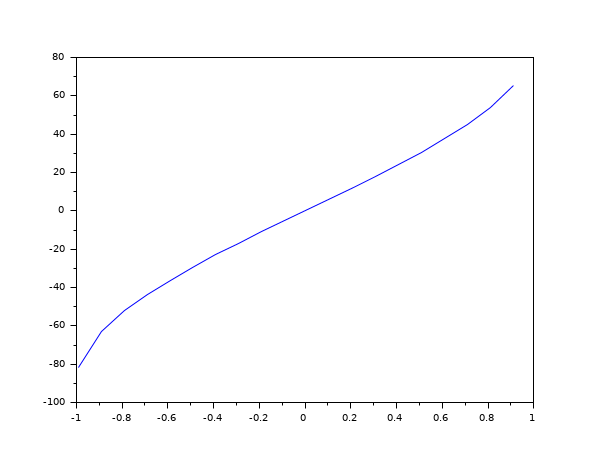Scilab Home page | Wiki | Bug tracker | Forge | Mailing list archives | ATOMS | File exchange
Change language to: Français - Português - 日本語 - Русский

# asind

sine inverse, results in degree

### Syntax

`t = asind(x)`

### Arguments

x

a real vector/matrix. Elements must be in `[-1 1]`.

t

a real vector/matrix with same dimensions as `x`

### Description

The entries of `t` are sine inverse of the corresponding entries of `x`. Definition domain is `[-1 1]`. The results are in `[-90 90]`.

### Sample### Examples

```x=[-1 0 1 sqrt(2)/2 -sqrt(2)/2 sqrt(3)/2 -sqrt(3)/2];
asind(x)```

• sin — sine function
• sind — sine function, argument in degree.
• sinm — matrix sine function
• asinm — matrix wise sine inverse